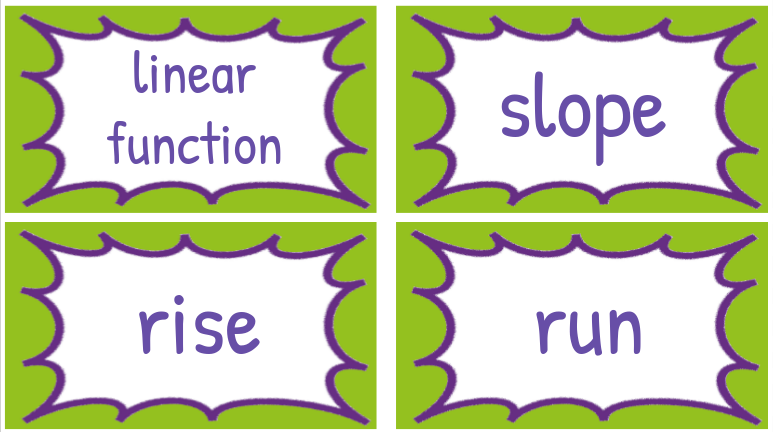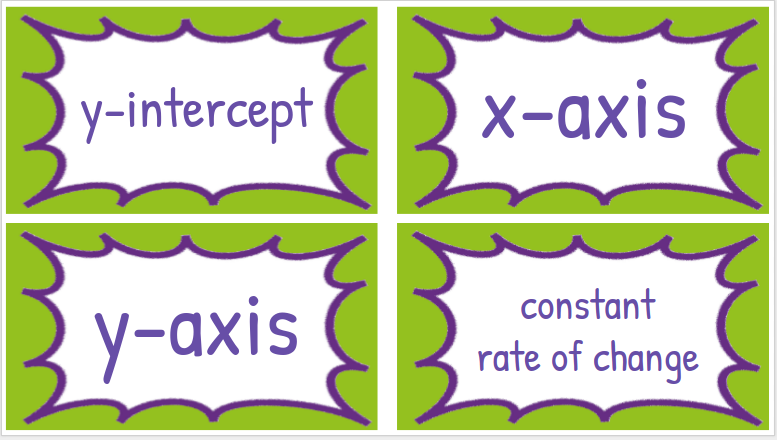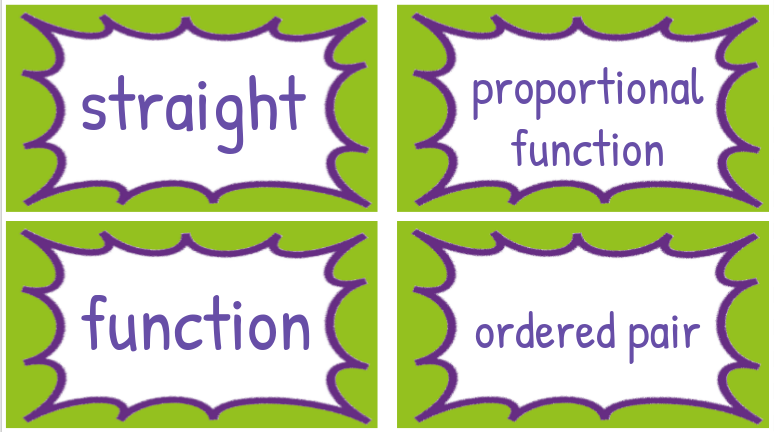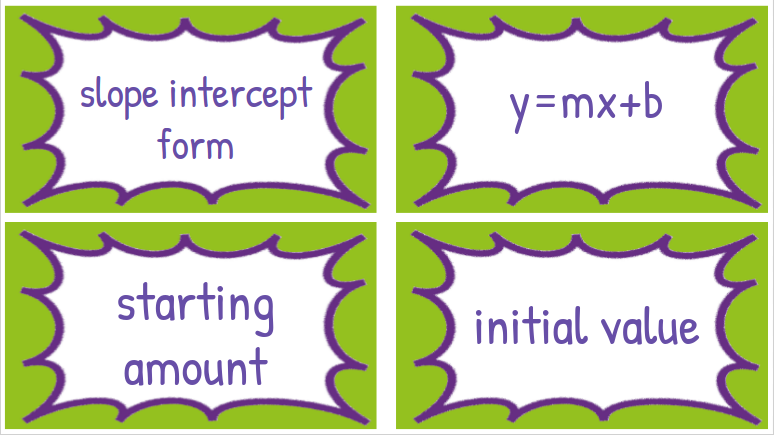## Monday, October 17, 2016

### Unit Vocab Cards: Part 1

Vocabulary Cards Set Up:

Every unit I make a list of vocabulary cards for that particular unit. This list includes on grade vocabulary for that unit as well as words and concepts that might have been taught in years previous, but still connect to my 8th grade unit.

The first unit for my 8th grade Pre-Algebra includes the following Common Core State Standards (as directly copied from the CCSS Grade 8 Standards found online here:

#### Define, evaluate, and compare functions.

CCSS.MATH.CONTENT.8.F.A.1
Understand that a function is a rule that assigns to each input exactly one output. The graph of a function is the set of ordered pairs consisting of an input and the corresponding output.1
CCSS.MATH.CONTENT.8.F.A.2
Compare properties of two functions each represented in a different way (algebraically, graphically, numerically in tables, or by verbal descriptions). For example, given a linear function represented by a table of values and a linear function represented by an algebraic expression, determine which function has the greater rate of change.
CCSS.MATH.CONTENT.8.F.A.3
Interpret the equation y = mx + b as defining a linear function, whose graph is a straight line; give examples of functions that are not linear. For example, the function A = s2giving the area of a square as a function of its side length is not linear because its graph contains the points (1,1), (2,4) and (3,9), which are not on a straight line.

#### Use functions to model relationships between quantities.

CCSS.MATH.CONTENT.8.F.B.4
Construct a function to model a linear relationship between two quantities. Determine the rate of change  and initial value of the function from a description of a relationship or from two (x, y) values, including reading these from a table or from a graph. Interpret the rate of change and initial value of a linear function in terms of the situation it models, and in terms of its graph or a table of values.
CCSS.MATH.CONTENT.8.F.B.5
Describe qualitatively the functional relationship between two quantities by analyzing a graph (e.g., where the function is increasing or decreasing, linear or nonlinear). Sketch a graph that exhibits the qualitative features of a function that has been described verbally.
Yikes. That's a lot, right? So from that list I created the following collection of vocabulary words in a Google Slide (found here) using some free fun template I found on the internet: